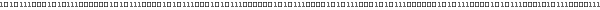# Lesson 8 : Tangent Space• ## Tangent Space theory

• ### What is Tangent Space and for which use ?

Tangent space is basicaly used for advanced rendering techniques such as normal mapping.
Briefly, normal mapping is a per pixel normal technique which is based on pixel's normal perturbation. Normals for each pixel are stored in as rgb picture called normal map. The normal is applied to the pixel in a similar way than for the color with the diffuse texture. The uv texture coordinate of the pixel is used for reading in the normal map for getting the pixel's normal. This is the normal used for light computation on this pixel (per-pixel lighting).
This normal is stored in this map in the tangent space coordinate system.

Tangent space is a coordinate system attached to a planar surface. The surface commonly used is a triangular shape. Tangent space transformation convert a vector from the world space into a vector in texture space.
In the above example, pixel normal is stored in texture space. Tangent space can be used to convert this normal in world space for light computation. Generaly, the inverse is done ie converting vectors used for light computation into tangent space ('texture' space) and do the lighting computation in texture space.

• ### Tangent Space definition

• #### Coordinate system definition

Tangent space is composed of 3 vectors (T, B, N) respectively called tangent, binormal and normal. Tangent and binormal are vectors in the plane. While normal vector is perpendicular to the place, so it is also perpendicular to tangent and binormal vectors.
In the texture space, (T, B) vectors correspond to (u, v) vectors. This means, T is the vector (1, 0) in texture space and B is the (0, 1). This is the coordinates of T and B in texture space.

You can see a representation of (T, B, N) coordinate system in both texture and world space. The picture wasFrom Matyas Premecz's paper 

The tangent space transformation convert a point expressed in world space ie (x, y, z) to a vector in texture space (u, v, w).
Tangent space is really usefull for advanced rendering texhnique which stores pixels information (normal, height, color ...) in a texture. Tangent space allow working directly in texture coordinate system

• #### Equation of T and B

Suppose a point pi in world coordinate system for which texture coordinates are (ui, vi), the relation between texture coordinates (ie ui ui, vi) and world space (pi) is govern by the relation .
Note: This point can also be considered as the vector starting from the origin to pi.

pi = ui.T + vi.B
Texture/World space relation

Writting this equation for the points p1, p2 and p3 give :

p1 = u1.T + v1.B
p2 = u2.T + v2.B
p3 = u3.T + v3.B

With equation manipulation (equation subtraction), we can write :

p2 - p1 = (u2 - u1).T + (v2 - v1).B
p3 - p1 = (u3 - u1).T + (v3 - v1).B

By resolving this system :

(v3 - v1).(p2 - p1)  =    (v3 - v1).(u2 - u1).T + (v3 - v1).(v2 - v1).B
- (v2 - v1).(p3 - p1)     - (v2 - v1).(u3 - u1).T - (v2 - v1).(v3 - v1).B

(u3 - u1).(p2 - p1)  =    (u3 - u1).(u2 - u1).T + (u3 - u1).(v2 - v1).B
- (u2 - u1).(p3 - p1)     - (u2 - u1).(u3 - u1).T - (u2 - u1).(v3 - v1).B

And we finally have the formula of T and B :

(v3 - v1).(p2 - p1) - (v2 - v1).(p3 - p1)
T = ---------------------------------------
(u2 - u1).(v3 - v1) - (v2 - v1).(u3 - u1)

(u3 - u1).(p2 - p1) - (u2 - u1).(p3 - p1)
B = ---------------------------------------
(v2 - v1).(u3 - u1) - (u2 - u1).(v3 - v1)

Equation of tangent and binormal vectors

• #### Equation of N

(T, B, N) is a base for the coordinate system. N can be obtained as the cross product of T by B :

N = cross(T, B)
Equation of normal vector

• #### Equation of T, B, N (Summary)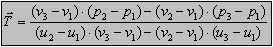Tangent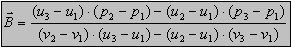Binormal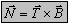Normal

• #### Properties

(T, B, N) form an orthonormal basis in texture space ie vectors are unit length and are all perpendicular between each other.
However, the transformation from the texture space into world space is not distance/angle conservative. So, generally, (T, B, N) is not orthonormal in world space. This means T and B are not necessarily perpendicular, but they are perpendicular with N. As well, these vectors are not necessary unitary.

• #### Transformation between world space and tangent space

The transformation matrix from is composed (T, B, N) vector in collumns :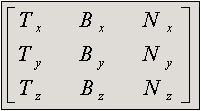TBN matrix

To transform a vector exprimed in world (object) space to a vector in tangent space, just multiply the matrix with the vector :

VWorld = TBN VTangent = VTangentT TBNT
Tangent space to world space

VTangent = TBN-1 VWorld = VWorldT TBN-T
World space to tangent space

Those expressions can written like this :

[x y z]T = TBN [u v w]T = [u v w] TBNT
Tangent space to world space

[u v w]T = TBN-1 [x y z]T = [x y z] TBN-T
World space to tangentspace

• ### Tangent Space implementation

The Java & GLSL code come from my Bonzai Engine project.
The Java source code uses some classes which is not detailled here, but I thinks that is easy understandable. The related documentation can be found in the Bonzai Engine project homepage.

Here is the java code that calculates to tangent, binormal and normal vector of a triangular face.
The Face object stores the definition of the traingle: the 3 vertices & their associated texture coordinates. The output is written to the 3 vectors tangent, binormal and normal vectors.

 Tangent, binormal and normal vectors of a triangle public static void computeFaceTBNBasis(/*Mesh mesh, */Face face, Vector3f tangent, Vector3f binormal, Vector3f normal) { //In case of using a Mesh, we use the commented code //    Vector3f p21  = subtract(mesh.vertices [face.vertexId   ], mesh.vertices [face.vertexId   ]); //    Vector3f p31  = subtract(mesh.vertices [face.vertexId   ], mesh.vertices [face.vertexId   ]); //    Vector2f uv21 = subtract(mesh.texCoords[face.texCoordsId], mesh.texCoords[face.texCoordsId]); //    Vector2f uv31 = subtract(mesh.texCoords[face.texCoordsId], mesh.texCoords[face.texCoordsId]);     Vector3f p21  = subtract(face.vertice], face.vertice]);  //p2-p1     Vector3f p31  = subtract(face.vertice], face.vertice]);  //p3-p1     Vector2f uv21 = subtract(face.uv],      face.uv]);       //uv2-uv1     Vector2f uv31 = subtract(face.uv],      face.uv]);       //uv3-uv1     /*float f = uv21.getX()*uv31.getY() - uv21.getY()*uv31.getX();     f = (f == 0) ? 1 : 1 / f;*/     //Note: As vectors are normalized, we can skeep the 1/f division and the normalization of the normal     //      Feel free to keep/enable the commented code.     if(tangent != null || normal != null)         tangent.copyFrom( normalize(/*multiply(*/multiply(p21, uv31.getY()).subtract(multiply(p31, uv21.getY()))/*, f)*/));     if(binormal != null || normal != null)         binormal.copyFrom(normalize(/*multiply(*/multiply(p31, uv21.getX()).subtract(multiply(p21, uv31.getX()))/*, f)*/));     if(normal != null)         normal.copyFrom( /*normalize(*/crossProduct(tangent, binormal)/*)*/); }

As noticed previously, the tangent and binormal vectors are not ensured to be perpendicular. Also, if normals are smoothed over faces, so the perpendicularity with tangent will be lost. Due to this, we do a Gram-Schmidt orthogonalization follow by a cross-product.

The orthogonalization is done between the tangent and normal vectors. Now, the binormal can be easily calculate with a cross product. Before doing this, we check if the TBN space is right or left handed. The TBN space can be left handed in the case of mirrored texture coordinates (see ... & the screenshot section). If the TBN space is left handed, we just invert the computed binormal to regenerate a left handed system.

 Orthogonalization of TBN space //Compute TBN vectors     Vector3f tangent = new Vector3f();     Vector3f binormal = new Vector3f();     //Note: mesh normals are already calculated (with normal smoothing).     //      For demonstration here, I use the normal calculated from computeFaceTBNBasis.     //     /*Vector3f normal = mesh.normals[face.normalId];     computeFaceTBNBasis(mesh, face, tangent, binormal, null);*/     Vector3f normal = new Vector3f();     computeFaceTBNBasis(mesh, face, tangent, binormal, normal );     //Gram-Schmidt orthogonalization     tangent.subtract(multiply(normal, dotProduct(normal, tangent))).normalize();     //Right handed TBN space ?     boolean rigthHanded = dotProduct(crossProduct(tangent, binormal), normal) >= 0;     binormal = crossProduct(normal, tangent);     if(!rigthHanded)         binormal.multiply(-1);

To use the TBN space in a GLSL Shader, first we have to send the vectors like shown underneath :

 Send Tangent space to GLSL shader /*      * Bind attributes      * ===============      * Before linking shader program with glLinkProgram,      * bind attributes name to a vertex index.      *      *      */     //Shader init (done one time): Bind attributes     gl.glBindAttribLocation(glsl.getProgram(), 12, "tangent");     gl.glBindAttribLocation(glsl.getProgram(), 13, "binormal");     //Sending tangent     gl.glVertexAttrib3f(12, tangent.getX(), tangent.getY(), tangent.getZ());     //Send binormal     gl.glVertexAttrib3f(13, binormal.getX(), binormal.getY(), binormal.getZ());     //Sending normal     gl.glNormal3f(normal.getX(), normal.getY(), normal.getZ());

Now, the TBN space in the GLSL shader code :

 GLSL Vertex Shader attribute vec3 tangent; attribute vec3 binormal; varying vec3 N; ... void main() {     //Normal in object space     N = (gl_NormalMatrix * gl_Normal).xyz;     //Tangent space matrix     //Note: normalize can be skiped as vectors are already normalized during tangent space creation,     //      the matrix multiplication does not change the vector amplitude.     //      So feel free to remove the 3 normalize calls.     vec3 t = normalize(gl_NormalMatrix * tangent);     vec3 b = normalize(gl_NormalMatrix * binormal);     vec3 n = normalize(N);     mat3 matrix = mat3(t, b, n);     //Convert normal in tangent space     N = N * matrix;     ...

• ## Screenshots

In the next screenshot, we can view the TBN space smoothed by vertex (TBN space averaged over adjacent faces).
Note: The TBN smoothing for right & left handed space are made separatly.

In the right side of the picture, the TBN space is right handed. The left side of the model, the texture is mirrored (ie with mirrored uv). Due to this, the TBN space is left handed. We can see the clear separation at the middle.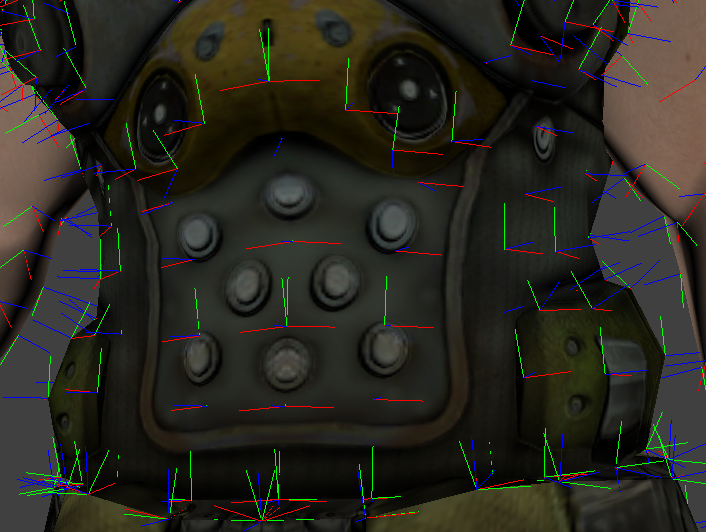TBN Space with Mirrored UV

T is displayed in red
B is displayed in green
N is displayed in blue

More screenshots in Project 4 : Shaders.

• ## References / Acknowledge

This article was written thanks to some really usefull and interesting informations from this paper. I strongly encourage you to read it.Next Lesson